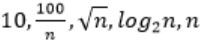October 12, 2023
October 12, 2023
October 12, 2023
###### GATE 2017 [Set-1]
October 12, 2023
 Question 4

Consider the following functions from positives integers to real numbersThe CORRECT arrangement of the above functions in increasing order of asymptotic complexity is:

 ABCDQuestion 4 Explanation:
In this problem, they are expecting to find us “increasing order of asymptotic complexity”.

Step-1: Take n=2048 or 211 (Always take n is very big number)
Step-2: Divide functions into 2 ways
1. Polynomial functions
2. Exponential functions
Step-3: The above functions are belongs to polynomial. So, simply substitute the value of n,
First compare with constant values.
→ 100 / 2048 = 0.048828125
→ 10 > 100/ 2048
→ log2 2048 =11
→ √n = 45.25483399593904156165403917471
→ n = 2048
So, Option B is correct
Question 4 Explanation:
In this problem, they are expecting to find us “increasing order of asymptotic complexity”.

Step-1: Take n=2048 or 211 (Always take n is very big number)
Step-2: Divide functions into 2 ways
1. Polynomial functions
2. Exponential functions
Step-3: The above functions are belongs to polynomial. So, simply substitute the value of n,
First compare with constant values.
→ 100 / 2048 = 0.048828125
→ 10 > 100/ 2048
→ log2 2048 =11
→ √n = 45.25483399593904156165403917471
→ n = 2048
So, Option B is correct